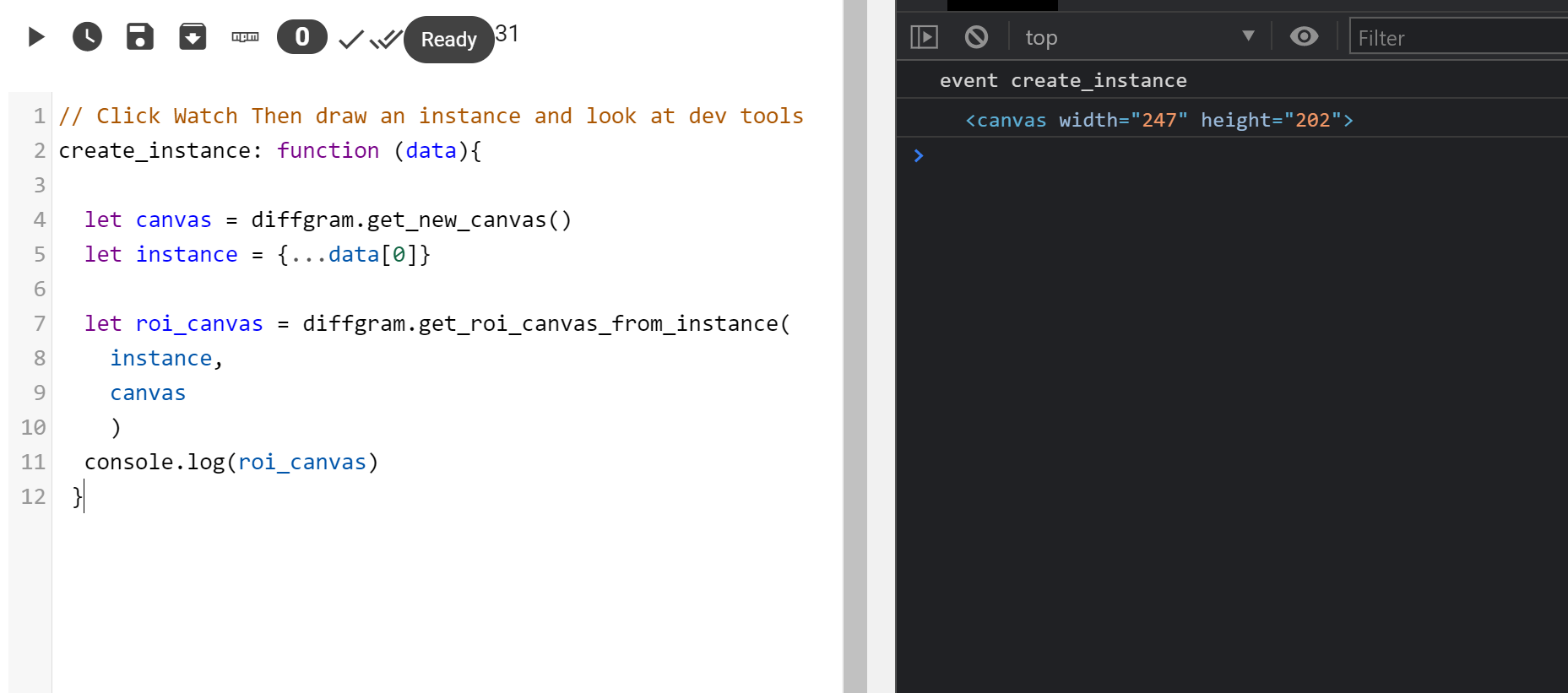# get_roi_canvas_from_instance

Get a Region of Interest (ROI) using an instance

`diffgram.get_roi_canvas_from_instance`

# Arguments

`instance` : a Diffgram instance
`canvas` : a `canvas` type element

# Description

The goal of this is to return a new canvas element ready to be consumed by functions that want to focus on a specific area of the image. For example grabcut or similar, or running an algorithm on a subsection of a large image or frame.

# Example

``````let canvas = diffgram.get_new_canvas()
let roi_canvas = diffgram.get_roi_canvas_from_instance(
instance,
canvas
)
``````

# Example (Using Watcher)

``````// Click Watch Then draw an instance and look at dev tools
create_instance: function (data){

let canvas = diffgram.get_new_canvas()
let instance = {...data}

let roi_canvas = diffgram.get_roi_canvas_from_instance(
instance,
canvas
)
console.log(roi_canvas)
}
``````

Drawing an instance example# Returns

A `canvas` cropped to the region desired